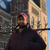# QlikView App Dev

Discussion Board for collaboration related to QlikView App Development.

Announcements
On May 18th at 10AM EDT we will answer your QlikView questions live. REGISTER
cancel
Showing results for
Did you mean:Creator II

## pls help

Hi,

I need help on ignoring selections.

In Qliksense, I have pie chart

with

dims-->Zone,counrty

and

measure--->Sum(distinct {<Year={"\$(=\$(_vCurrentYear))"},Brand=>}volume)

My requirement is : If I select any country in pie chart, it shouldn't be effect in other chart(bar chart)

In Bar chart

dim--->Country&'-'&Brand

and

Measure1--->

(((Sum({1<Measure=,[KPI Type]={"Volume"},KPI={"B F C"},

BF_Year={"\$(=\$(_vBFCurrentYear))"}>}BF_Volume))/1000)

/

((Sum({1<Measure=,[KPI Type]={"Volume"},KPI={"B F C"},

BF_Year={"\$(=\$(_vBFLastYear))"}>}BF_Volume))/1000)

-1)

*100

Measure2--->

(Sum({1<Measure=,[KPI Type]={"Volume"},KPI={"B F C"},

BF_Year={"\$(=\$(_vBFCurrentYear))"},Country>}BF_Volume))/1000

I tried with Country= under the set expression but not working

any suggestions???

9 RepliesChampion

Hi,

it is possible to attach a sample QVW with this problem?MVP

The question is posted in a QlikView forum, but I don't think it is a QlikView question...Creator II
Author

hey thanks  for your quick response!

not able share the application as am working with real data.

Can you suggest me  on the above expressions where I did wrong???Creator II
Author

Yes its Qliksense question.

unfortunately I posted in Qlikview forum later on I came to know this.

That's why I posted in Qliksense forum.Partner

Since is a QlikView app, and assuming that is correct information, you could use Alternate states and put the pie chart in an alternate, which would then prevent selections made within the chart to influence other charts.

If Alternate States doesn't work, you can try to make the chart Read-only, or maybe populate that chart with data from a data island, rather than the data model.

Good luck and let us know if those suggestions help.

NateMVP

No problem. You can always move a dsicussion you created to another forum if you feel this is more appropriate.ChampionCreator II
Author

_vBFCurrentYear= Only([Fc St Yr])-1 and result is 2017

_vBFLastYear= Num(Only([Fc St Yr])-2,'####') and result is 2016

I think problem with variables

If I hard-code 2016 and 2017 in the below exp am getting correct what exactly looking for.

(((Sum({1<Measure=,[KPI Type]={"Volume"},KPI={"B F C"},

BF_Year={"\$(=\$(_vBFCurrentYear))"}>}BF_Volume))/1000)

/

((Sum({1<Measure=,[KPI Type]={"Volume"},KPI={"B F C"},

BF_Year={"\$(=\$(_vBFLastYear))"}>}BF_Volume))/1000)

-1)

*100

Where I did wrong with the variables??

Pls suggestMaster III

May be try

BF_Year={"\$(=\$(_vBFCurrentYear))"}

as

BF_Year={'\$(=_vBFCurrentYear)'}